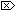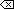The Open Group Base Specifications Issue 6
IEEE Std 1003.1-2001

#### SYNOPSIS

```[OB XSI]#include <math.h> double scalb(double x, double n);```

#### DESCRIPTION

The scalb() function shall compute x*rn, where r is the radix of the machine's floating-point arithmetic. When r is 2, scalb() shall be equivalent to ldexp() . The value of r is FLT_RADIX which is defined in <float.h>.

An application wishing to check for error situations should set errno to zero and call feclearexcept(FE_ALL_EXCEPT) before calling these functions. On return, if errno is non-zero or fetestexcept(FE_INVALID | FE_DIVBYZERO | FE_OVERFLOW | FE_UNDERFLOW) is non-zero, an error has occurred.

#### RETURN VALUE

Upon successful completion, the scalb() function shall return x*rn.

If x or n is NaN, a NaN shall be returned.

If n is zero, x shall be returned.

If x is ±Inf and n is not -Inf, x shall be returned.

If x is ±0 and n is not +Inf, x shall be returned.

If x is ±0 and n is +Inf, a domain error shall occur, and either a NaN (if supported), or an implementation-defined value shall be returned.

If x is ±Inf and n is -Inf, a domain error shall occur, and either a NaN (if supported), or an implementation-defined value shall be returned.

If the result would cause an overflow, a range error shall occur and ±HUGE_VAL (according to the sign of x) shall be returned.

If the correct value would cause underflow, and is representable, a range error may occur and the correct value shall be returned.

If the correct value would cause underflow, and is not representable, a range error may occur, and 0.0 shall be returned.

#### ERRORS

The scalb() function shall fail if:

Domain Error
If x is zero and n is +Inf, or x is Inf and n is -Inf.

If the integer expression (math_errhandling & MATH_ERRNO) is non-zero, then errno shall be set to [EDOM]. If the integer expression (math_errhandling & MATH_ERREXCEPT) is non-zero, then the invalid floating-point exception shall be raised.

Range Error
The result would overflow.

If the integer expression (math_errhandling & MATH_ERRNO) is non-zero, then errno shall be set to [ERANGE]. If the integer expression (math_errhandling & MATH_ERREXCEPT) is non-zero, then the overflow floating-point exception shall be raised.

The scalb() function may fail if:

Range Error
The result underflows.

If the integer expression (math_errhandling & MATH_ERRNO) is non-zero, then errno shall be set to [ERANGE]. If the integer expression (math_errhandling & MATH_ERREXCEPT) is non-zero, then the underflow floating-point exception shall be raised.

The following sections are informative.

None.

#### APPLICATION USAGE

Applications should use either scalbln(), scalblnf(), or scalblnl() in preference to this function.

IEEE Std 1003.1-2001 only defines the behavior for the scalb() function when the n argument is an integer, a NaN, or Inf. The behavior of other values for the n argument is unspecified.

On error, the expressions (math_errhandling & MATH_ERRNO) and (math_errhandling & MATH_ERREXCEPT) are independent of each other, but at least one of them must be non-zero.

None.

#### FUTURE DIRECTIONS

None.

feclearexcept() , fetestexcept() , ilogb() , ldexp() , logb() , scalbln() , the Base Definitions volume of IEEE Std 1003.1-2001, Section 4.18, Treatment of Error Conditions for Mathematical Functions, <float.h>, <math.h>

#### CHANGE HISTORY

First released in Issue 4, Version 2.

#### Issue 5

Moved from X/OPEN UNIX extension to BASE.

The DESCRIPTION is updated to indicate how an application should check for an error. This text was previously published in the APPLICATION USAGE section.

#### Issue 6

This function is marked obsolescent.

Although this function is not part of the ISO/IEC 9899:1999 standard, the RETURN VALUE and ERRORS sections are updated to align with the error handling in the ISO/IEC 9899:1999 standard.

End of informative text.

UNIX ® is a registered Trademark of The Open Group.
POSIX ® is a registered Trademark of The IEEE.
[ Main Index | XBD | XCU | XSH | XRAT ]